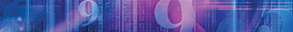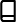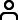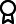Journal metrics
See full report
Acceptance rate39%
Submission to final decision58 days
Acceptance to publication20 days
CiteScore1.500
Journal Citation Indicator0.490
Impact Factor1.364

### Abundant Soliton Structures to the ()-Dimensional Heisenberg Ferromagnetic Spin Chain Dynamical Model

####Journal profile

Advances in Mathematical Physics publishes papers that seek to understand mathematical basis of physical phenomena, and solve problems in physics via mathematical approaches.

####Editor spotlight

Chief Editor, Prof Di Matteo (Department of Mathematics, King’s College London), engages in world-leading multidisciplinary and data-driven research focussed on the analysis of complex data from the perspective of a statistical physicist.

####Special Issues

Do you think there is an emerging area of research that really needs to be highlighted? Or an existing research area that has been overlooked or would benefit from deeper investigation? Raise the profile of a research area by leading a Special Issue.

## Latest Articles

More articles
Research Article

### Exact Solution of ()-Dimensional Boiti–Leon–Manna–Pempinelli Equation

Based on the Hirota bilinear method, using the heuristic function method and mathematical symbolic computation system, various exact solutions of the ()-dimensional Boiti–Leon–Manna–Pempinelli equation including the block kink wave solution, block soliton solution, periodic block solution, and new composite solution are obtained. Upon selection of the appropriate parameters, three-dimensional and contour diagrams of the exact solution were generated to illustrate their properties.

Research Article

### Lattice Boltzmann Method for the Generalized Black-Scholes Equation

In this paper, an efficient lattice Boltzmann model for the generalized Black-Scholes equation governing option pricing is proposed. The Black-Scholes equation is firstly equivalently transformed into an initial value problem for a partial differential equation with a source term using the variable substitution and the derivative rules, respectively. Then, applying the multiscale Chapman-Enskog expansion, the amending function is expanded to second order to recover the convective and source terms of the macroscopic equation. The D1Q3 lattice Boltzmann model with spatial second-order accuracy is constructed, and the accuracy of the established D1Q5 model is greater than second order. The numerical simulation results demonstrate the effectiveness and numerical accuracy of the proposed models and indicate that our proposed models are suitable for solving the Black-Scholes equation. The proposed lattice Boltzmann model can also be applied to a class of partial differential equations with variable coefficients and source terms.

Research Article

### Interaction Solutions of the (2+1)-Dimensional Sawada-Kotera Equation

The N-soliton solution of the (2+1)-dimensional Sawada-Kotera equation is given by using the Hirota bilinear method, and then, the conjugate parameter method and the long-wave limit method are used to get the breather solution and the lump solution, as well as the interaction solution of the elastic collision properties between them. In addition, according to the expression of the lump-type soliton solution and the striped soliton solution, the completely inelastic collision, rebound, absorption, splitting, and other particle characteristics of the two solitons in the interaction process are directly studied with the simulation method, which reveals the laws of physics reflected behind the phenomenon.

Research Article

### Dynamical Property of the Shift Map under Group Action

Firstly, we introduced the concept of Lipschitz tracking property, asymptotic average tracking property, and periodic tracking property. Secondly, we studied their dynamical properties and topological structure and obtained the following conclusions: (1) let be compact metric space and the metric be invariant to . Then, has asymptotic average tracking property; (2) let be compact metric space and the metric be invariant to . Then, has Lipschitz tracking property; (3) let be compact metric space and the metric be invariant to . Then, has periodic tracking property. The above results make up for the lack of theory of Lipschitz tracking property, asymptotic average tracking property, and periodic tracking property in infinite product space under group action.

Research Article

### Wave Breaking and Global Existence for the Generalized Periodic Camassa-Holm Equation with the Weak Dissipation

In this paper, a family of the weakly dissipative periodic Camassa-Holm type equation cubic and quartic nonlinearities is considered. The precise blow-up scenarios of strong solutions and several conditions on the initial data to guarantee blow-up of the induced solutions are described in detail. Finally, we establish a sufficient condition for global solutions.

Research Article

### Optical Wave Phenomena in Birefringent Fibers Described by Space-Time Fractional Cubic-Quartic Nonlinear Schrödinger Equation with the Sense of Beta and Conformable Derivative

The work explores the optical wave solutions along with their graphical representations by proposing the coupled spatial-temporal fractional cubic-quartic nonlinear Schrödinger equation with the sense of two fractal derivatives (beta and conformable derivative) and Kerr law nonlinearity for birefringent fibers. The new extended direct algebraic method for the first time is implemented to achieve this goal. Many optical solutions are listed along with their existence criteria. Based on the existence criteria, the cubic-quartic bright, and singular optical soliton, periodic pulse, and rouge wave profiles are supported in birefringent fibers with the influence of both beta and conformable derivative parameter.Journal metrics
See full report
Acceptance rate39%
Submission to final decision58 days
Acceptance to publication20 days
CiteScore1.500
Journal Citation Indicator0.490
Impact Factor1.364Author guidelinesEditorial boardDatabases and indexingAuthor guidelinesEditorial boardDatabases and indexing

Article of the Year Award: Outstanding research contributions of 2021, as selected by our Chief Editors. Read the winning articles.